JEE  >  Part Test - 14 (JEE Advanced)

# Part Test - 14 (JEE Advanced)

Test Description

## 60 Questions MCQ Test | Part Test - 14 (JEE Advanced)

Part Test - 14 (JEE Advanced) for JEE 2023 is part of JEE preparation. The Part Test - 14 (JEE Advanced) questions and answers have been prepared according to the JEE exam syllabus.The Part Test - 14 (JEE Advanced) MCQs are made for JEE 2023 Exam. Find important definitions, questions, notes, meanings, examples, exercises, MCQs and online tests for Part Test - 14 (JEE Advanced) below.
Solutions of Part Test - 14 (JEE Advanced) questions in English are available as part of our course for JEE & Part Test - 14 (JEE Advanced) solutions in Hindi for JEE course. Download more important topics, notes, lectures and mock test series for JEE Exam by signing up for free. Attempt Part Test - 14 (JEE Advanced) | 60 questions in 180 minutes | Mock test for JEE preparation | Free important questions MCQ to study for JEE Exam | Download free PDF with solutions
 1 Crore+ students have signed up on EduRev. Have you?
Part Test - 14 (JEE Advanced) - Question 1

### The amount of heat evolved when 500 cm3 of 0.1 M HCl is mixed with 200 cm3 of 0.2 M NaoH is

Part Test - 14 (JEE Advanced) - Question 2

### A graph of the x component of the electric field as a function of x in a region of space is shown. The Y and Z components of the electric field are zero in this region. If the electric potential is 10 V at the origin, then potential at x = 2.0 m is :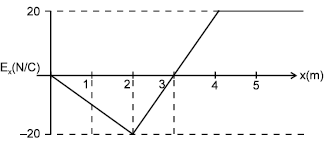Detailed Solution for Part Test - 14 (JEE Advanced) - Question 2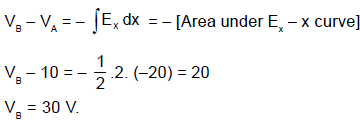Part Test - 14 (JEE Advanced) - Question 3

### A metallic charged ring is placed in a uniform magnetic field with its plane perpendicular to the field. If the magnitude of field starts increasing with time, then :

Detailed Solution for Part Test - 14 (JEE Advanced) - Question 3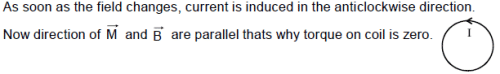Part Test - 14 (JEE Advanced) - Question 4

The empirical formula of a nonelectrolyte is CH2O. A solution containing 3g of the compound exerts the same osmotic pressure as that of 0.05 M glucose solution. The molecular formula of the compound is

Part Test - 14 (JEE Advanced) - Question 5

The mass of glucose that should be dissolved in 50 g of water in order to produce the same lowering of vapour pressure as is produced by dissolving 1 g of urea in the same quantity of water is

Part Test - 14 (JEE Advanced) - Question 6

A bat flies at a steady speed of 4 ms-1 emitting a sound of f = 90 ´ 10Hz. It is flying horizontally towards a vertical wall. The frequency of the reflected sound as detected by the bat will be _____.

(Take velocity of sound in air as 330 ms-1).

Part Test - 14 (JEE Advanced) - Question 7

A battery of e.m.f. E has an internal resistance 'r'. A variable resistance R is connected to the terminals of the battery. A current I is drawn from the battery. V is the terminal P.D. lf R alone is gradually reduced to zero, which of the following best describes I and V?

Part Test - 14 (JEE Advanced) - Question 8

A beam of light of wavelength 600 nm from a distant source falls on a single slit 1mm wide and the resulting diffraction pattern is observed on a screen 2m away. The distance between the first dark fringes on either side of the central bright fringe is _______.

Part Test - 14 (JEE Advanced) - Question 9

A biconvex lens has a radius of curvature of magnitude 20 cm. Which one of the following options describe best the image formed of an object of height 2 cm placed 30 cm from the lens?

Part Test - 14 (JEE Advanced) - Question 10

A plano-concave lens is made of glass of refractive index 1.5 and the radius of curvature of its curved face is 100 cm. What is the power of the lens?

Part Test - 14 (JEE Advanced) - Question 11

Current 'I' is flowing in a conductor shaped as shown in the figure. The radius of the curved part is r and the length of straight portion is very large. The value of the magnetic field at the centre O will be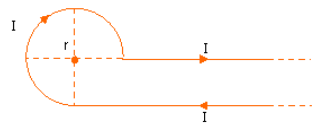Part Test - 14 (JEE Advanced) - Question 12

Two beams of red and violet colours are made to pass separately through a prism of A = 60°. In the minimum deviation position, the angle of refraction inside the prism will be

*Multiple options can be correct
Part Test - 14 (JEE Advanced) - Question 13

At distance of 5cm and 10cm outwards from the surface of a uniformly charged solid sphere, the potentials are 100V and 75V respectively. Then

Detailed Solution for Part Test - 14 (JEE Advanced) - Question 13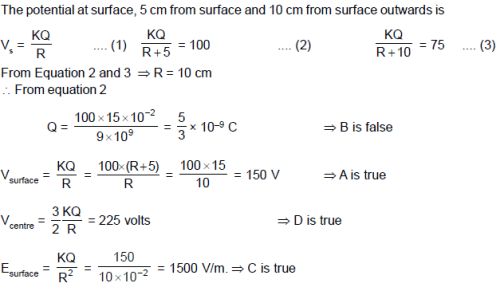*Multiple options can be correct
Part Test - 14 (JEE Advanced) - Question 14

A conducting rod of length l is moved at constant velocity ‘v0’ on two parallel, conducting, smooth, fixed rails, that are placed in a uniform constant magnetic field B perpendicular to the plane of the rails as shown in figure. A resistance R is connected between the two ends of the rail. Then which of the following is are correct :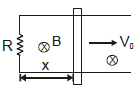Detailed Solution for Part Test - 14 (JEE Advanced) - Question 14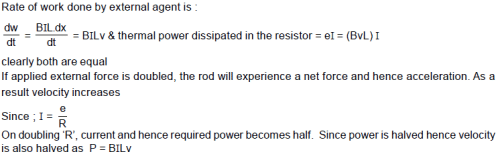Part Test - 14 (JEE Advanced) - Question 15

A battery of e.m.f. E has an internal resistance 'r'. A variable resistance R is connected to the terminals of the battery. A current I is drawn from the battery. V is the terminal P.D. lf R alone is gradually reduced to zero, which of the following best describes I and V?

*Answer can only contain numeric values
Part Test - 14 (JEE Advanced) - Question 16

In the circuit shown, current through the resistance 2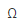is i1 and current through the resistance 30is i2. Find the ratio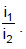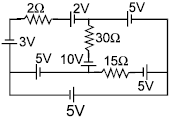Detailed Solution for Part Test - 14 (JEE Advanced) - Question 16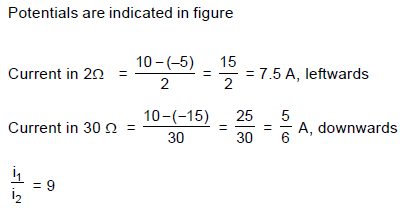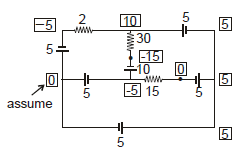*Answer can only contain numeric values
Part Test - 14 (JEE Advanced) - Question 17

In the figure shown the switch S1 remains connected for long time and the switch S2 remains opened. Now the switch S2 is closed. Assuming that ε = 10 volt and L = 1H,  the magnitude of  rate of change of current is 2X (in Amp/sec.) in the inductor just after the switch S2 is closed then find X .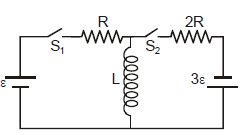Detailed Solution for Part Test - 14 (JEE Advanced) - Question 17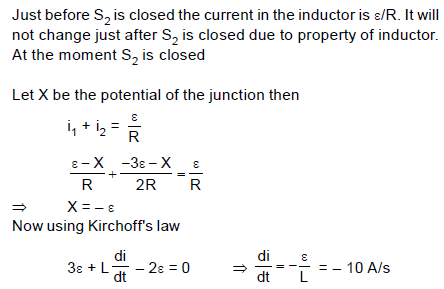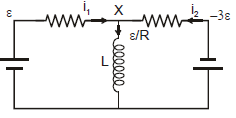*Answer can only contain numeric values
Part Test - 14 (JEE Advanced) - Question 18

A point source of radiation power P is placed on the axis of completely absorbing disc. The distance between the source and the disc is 2 times the radius of the disc. The force that light exerts on the disc is P/4ac then find a (c = speed of light).

Detailed Solution for Part Test - 14 (JEE Advanced) - Question 18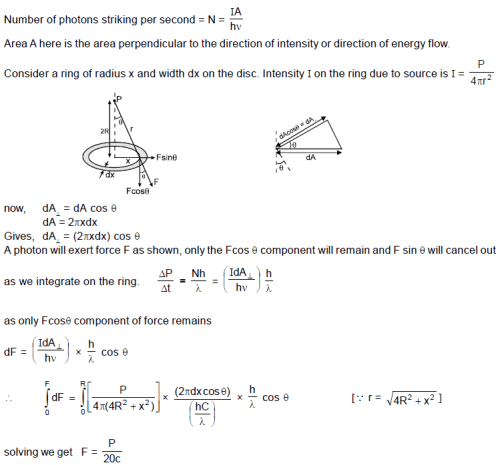*Answer can only contain numeric values
Part Test - 14 (JEE Advanced) - Question 19

In the figure, a conducting rod of length  l = 1 meter and mass m = 1 kg moves with initial velocity u = 5 m/s. on a fixed horizontal frame containing inductor L = 2 H and resistance R = 1. PQ and MN are smooth, conducting wires. There is a uniform magnetic field of strength B = 1T. Initially there is no current in the inductor. Find the total charge in coulomb, flown through the inductor by the time velocity of rod becomes vf = 1 m/s and the rod has travelled a distance x= 3 meter.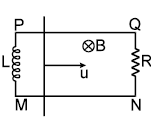Detailed Solution for Part Test - 14 (JEE Advanced) - Question 19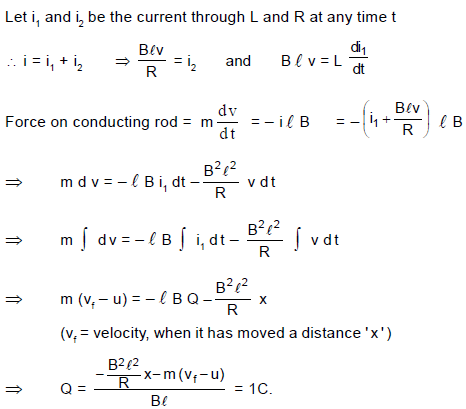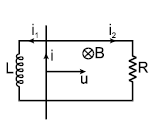*Answer can only contain numeric values
Part Test - 14 (JEE Advanced) - Question 20

In a YDSE arrangement composite lights of different wavelengths λ1 = 560 nm and λ2 = 400 nm are used. If D = 1m, d = 0.1 mm. If the distance between two nearest completely dark regions is  7X mm then X is :

Detailed Solution for Part Test - 14 (JEE Advanced) - Question 20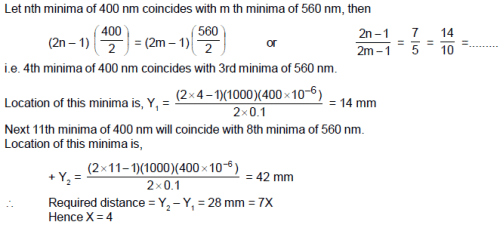Part Test - 14 (JEE Advanced) - Question 21

A unknown liquid has vapour pressure of  76 torr at temperature of 270C. If the enthalpy of vaporization of liquid is 5.52 kcal/mole, then the normal boiling point of liquid will be :  [Take ln10 = 2.3 ; R = 2 cal/mole-K]

Detailed Solution for Part Test - 14 (JEE Advanced) - Question 21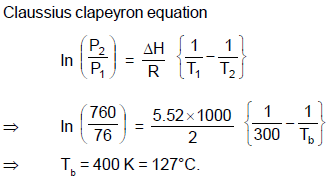Part Test - 14 (JEE Advanced) - Question 22

For a first order reaction, nA → B whose concentration vs time curve is as shown in the figure. If half-life for this reaction is 24 minutes. Find out the value of n.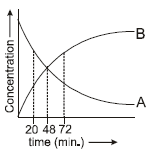Detailed Solution for Part Test - 14 (JEE Advanced) - Question 22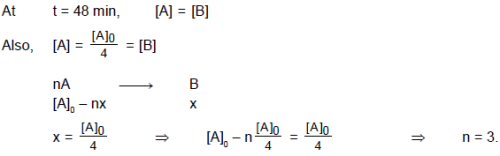Part Test - 14 (JEE Advanced) - Question 23

A body of mass 4 kg is accelerated upon by a constant force, travels a distance of 5 m in the first second and a distance of 2 m in the third second. The force acting on the body is

Part Test - 14 (JEE Advanced) - Question 24

In the zinc blende structure, zinc ions occupy alternate tetrahedral voids and S2–  ions exist as ccp the radii of Zn2+ and S2– ions are 0.83 Å and 1.74 Å respectively. The edge length of the ZnS unit cell is :

Detailed Solution for Part Test - 14 (JEE Advanced) - Question 24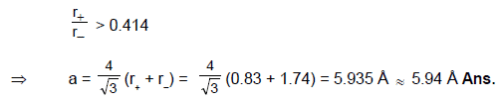Part Test - 14 (JEE Advanced) - Question 25

Which of the following statement(s) is (are) incorrect ?

Detailed Solution for Part Test - 14 (JEE Advanced) - Question 25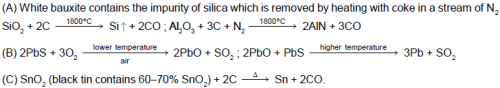Part Test - 14 (JEE Advanced) - Question 26

A 0.1 M solution of a certain cation will form a precipitate with 0.1 M solution of all these anions ;
OH, CO32–, Cl , SO42–. Which cation fits this description ?

Detailed Solution for Part Test - 14 (JEE Advanced) - Question 26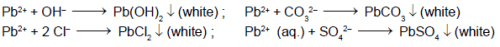Part Test - 14 (JEE Advanced) - Question 27

Which one of the following statements is incorrect ?

Detailed Solution for Part Test - 14 (JEE Advanced) - Question 27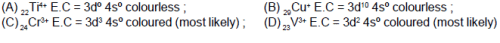Part Test - 14 (JEE Advanced) - Question 28

A closed organ pipe and an open organ pipe have their first overtones identical in frequency. Their lengths are in the ratio of

Part Test - 14 (JEE Advanced) - Question 29

A person of mass 60 kg is inside a lift of mass 940 kg and presses the button one control panel. The lift starts moving upwards with an acceleration 1.0 m/s2. If g = 10 ms–2, the tension in the supporting cable is

Part Test - 14 (JEE Advanced) - Question 30

If Avogadro number NA, is changed from 6.022 × 1023 mol–1 to 6.022 × 1020 mol–1, this would change

*Multiple options can be correct
Part Test - 14 (JEE Advanced) - Question 31

Dry air is slowly passed through three solutions of  different concentrations, c1, c2 and c3 ; each containing (non volatile) NaCl as solute and water as solvent, as shown in  the Figure If the vessel - 2 gains weight and the vessel 3 loses weight, then :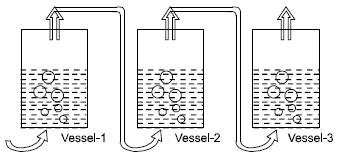Detailed Solution for Part Test - 14 (JEE Advanced) - Question 31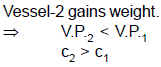Part Test - 14 (JEE Advanced) - Question 32

Concentrated aqueous sulphuric acid is 98% H2SO4 by mass and has a density of 1.80 g.mL–1. Volume of acid required to make 1 litre of 0.1 M H2SO4 solution is:

*Multiple options can be correct
Part Test - 14 (JEE Advanced) - Question 33

Which of the following statement(s) is (are) correct for the thiosulphate salt ?

Detailed Solution for Part Test - 14 (JEE Advanced) - Question 33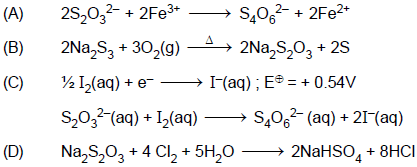*Multiple options can be correct
Part Test - 14 (JEE Advanced) - Question 34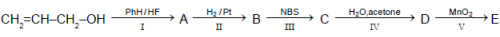Which of the following statements is/are correct ?

Detailed Solution for Part Test - 14 (JEE Advanced) - Question 34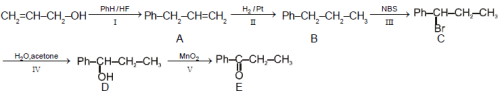*Multiple options can be correct
Part Test - 14 (JEE Advanced) - Question 35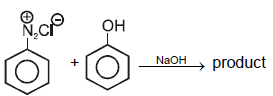Which of the following statements is/are correct about the above reaction ?

Detailed Solution for Part Test - 14 (JEE Advanced) - Question 35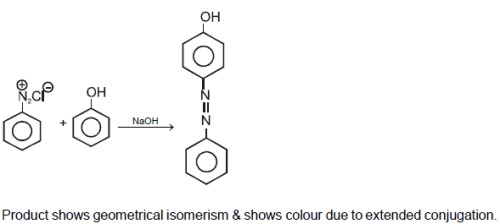*Answer can only contain numeric values
Part Test - 14 (JEE Advanced) - Question 36

The familiar brown ring test for nitrates depends on the ability of Fe2+ to reduce nitrates to nitric oxide, which reacts with Fe2+ to form a brown coloured complex [Fe(H2O)5NO+]SO4. What is the oxidation state of iron in the complex. (Only write the number 1, 2, 3, ......)

Detailed Solution for Part Test - 14 (JEE Advanced) - Question 36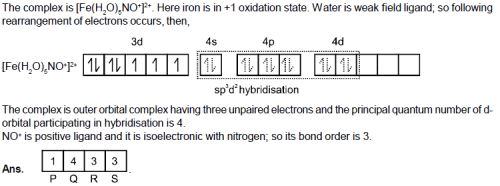*Answer can only contain numeric values
Part Test - 14 (JEE Advanced) - Question 37

Determine the number of unpaired electrons in the Cr3+ gaseous ions.

Detailed Solution for Part Test - 14 (JEE Advanced) - Question 37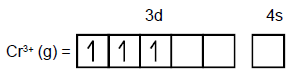*Answer can only contain numeric values
Part Test - 14 (JEE Advanced) - Question 38

In the following sequence of reactions all stereoisomers of (X) have been taken.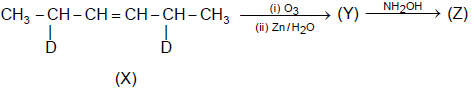Find the Total number of products (Z) formed.

Detailed Solution for Part Test - 14 (JEE Advanced) - Question 38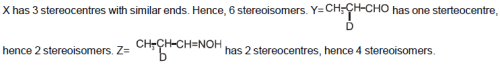*Answer can only contain numeric values
Part Test - 14 (JEE Advanced) - Question 39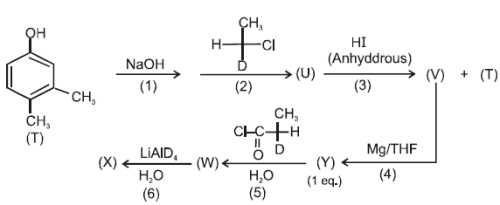Observe the following reaction sequence carefully.

Find  the total number of stereoisomers formed in step (6).

Detailed Solution for Part Test - 14 (JEE Advanced) - Question 39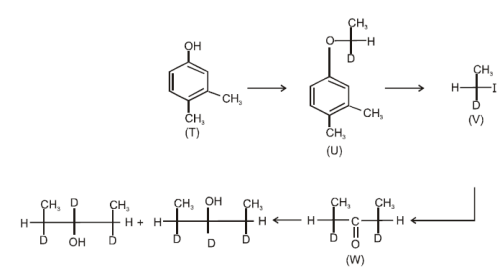*Answer can only contain numeric values
Part Test - 14 (JEE Advanced) - Question 40

1 g of a monobasic acid dissolved in 200 g of water lowers the freezing point by 0.186ºC. On the other hand when  1 g of the same acid is dissolved in water so as to make the solution 200 mL, this solution requires 125 mL of 0.1 N NaOH for complete neutralization. If α for the acid is x × 10. Find x :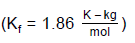Detailed Solution for Part Test - 14 (JEE Advanced) - Question 40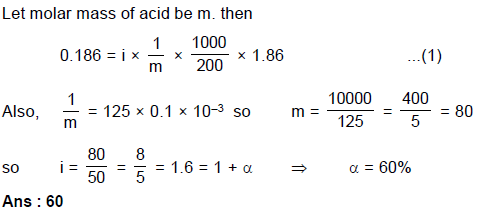Part Test - 14 (JEE Advanced) - Question 41

Number of moles of MnO4 required to oxidize one mole of ferrous oxalate completely in acidic medium will be

Part Test - 14 (JEE Advanced) - Question 42

If the change in the value of g at the height h above the surface of the earth is the same as at a depth x below it, then (both x and h being much smaller than the radius of the earth)

Part Test - 14 (JEE Advanced) - Question 43

The value of ‘g’ at a particular point is 9.8 m/sec2 suppose the earth suddenly shrink uniformly to half its present size without losing any mass. The value of ‘g’ at the same point (assuming that the distance of the point from the centre of the earth does not shrink) will become

Part Test - 14 (JEE Advanced) - Question 44

If x + 2 is a factor of x3 – 2ax2 + 16, then value of a is

Part Test - 14 (JEE Advanced) - Question 45

A body weighs 50 grams in air and 40 grams in water. How much would it weigh in a liquid of specific gravity 1.5?

Part Test - 14 (JEE Advanced) - Question 46

If A class has 175 students . The following data shows the number of students offering one or more subjects. Mathematics 100 ; Physics 70 ; Chemistry 40 ; Mathematics and Physics 30 ; Mathematics and Chemistry 28 ; Physics and Chemistry 23 ; Mathematics , Physics and Chemistry 18 . How many students have offered Mathematics alone ?

Part Test - 14 (JEE Advanced) - Question 47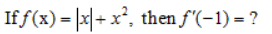Part Test - 14 (JEE Advanced) - Question 48

If A is a diagonal matrix of order ‘n’ such that A3A–1 = A, then number of possible values of matrix A, is

Detailed Solution for Part Test - 14 (JEE Advanced) - Question 48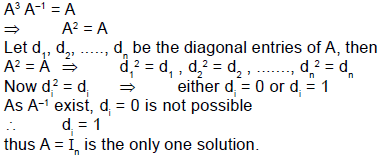Part Test - 14 (JEE Advanced) - Question 49

If f(x) = x + ex, and g(x) is the inverse function of f(x), then g``(A) equals

Detailed Solution for Part Test - 14 (JEE Advanced) - Question 49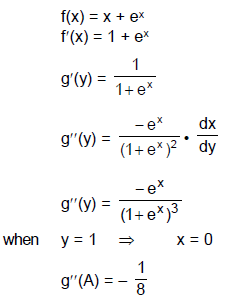Part Test - 14 (JEE Advanced) - Question 50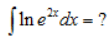*Multiple options can be correct
Part Test - 14 (JEE Advanced) - Question 51

If f (x) = x + |x| + cos ([π2] x) and g (x) = sin x then ([.] denotes greatest integer function) :

Detailed Solution for Part Test - 14 (JEE Advanced) - Question 51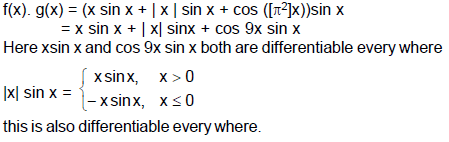Part Test - 14 (JEE Advanced) - Question 52

Two beams of red and violet colours are made to pass separately through a prism of A = 60°. In the minimum deviation position, the angle of refraction inside the prism will be

Part Test - 14 (JEE Advanced) - Question 53

If one of the factor of x2 + x – 20 is (x + 5). Find the other

Part Test - 14 (JEE Advanced) - Question 54

Three-fourth of the number of girls in a school is equal to half of the number of boys. If the school has 1420 pupils, how many of them are boys ?

Part Test - 14 (JEE Advanced) - Question 55

Compare the statements A and B.

Statement A: Synthesis of DNA takes place in the S-phase of interphase.

Statement B: Every chromosome, during metaphase, has two chromatids.

Choose the correct description :

*Answer can only contain numeric values
Part Test - 14 (JEE Advanced) - Question 56

Find the volume of the tetrahedron, if lengths of two opposite sides are 2 and 4 respectively and the shortest distance between them is 6 and these sides are inclined at 30º.

Detailed Solution for Part Test - 14 (JEE Advanced) - Question 56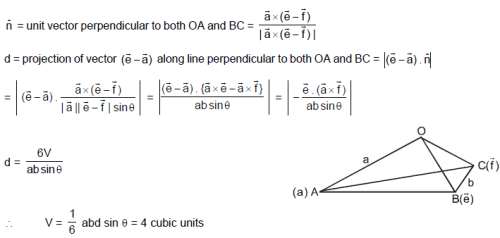*Answer can only contain numeric values
Part Test - 14 (JEE Advanced) - Question 57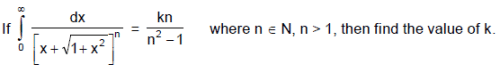Detailed Solution for Part Test - 14 (JEE Advanced) - Question 57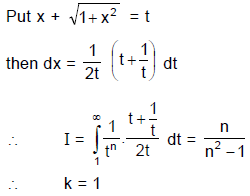*Answer can only contain numeric values
Part Test - 14 (JEE Advanced) - Question 58

The position vectors of two points A and C are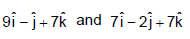respectively. The point of
intersection of the lines containing vectors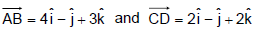is P. If vector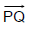is
perpendicular to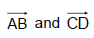and PQ = 15 units, then possible position vectors of Q are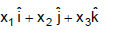and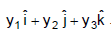Find the value ofDetailed Solution for Part Test - 14 (JEE Advanced) - Question 58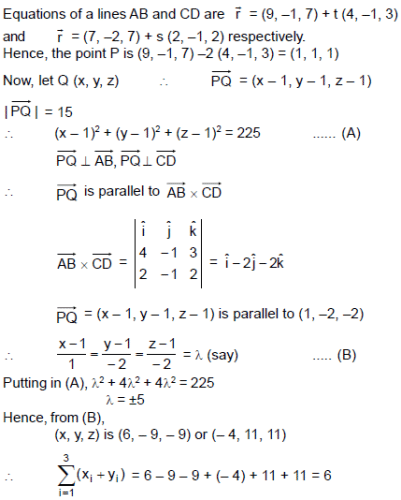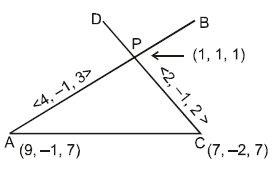*Answer can only contain numeric values
Part Test - 14 (JEE Advanced) - Question 59

Find the smallest positive integer 'p' for which the equation cos (p sinx) = sin (p cosx) has a solution in [0, 2π].

Detailed Solution for Part Test - 14 (JEE Advanced) - Question 59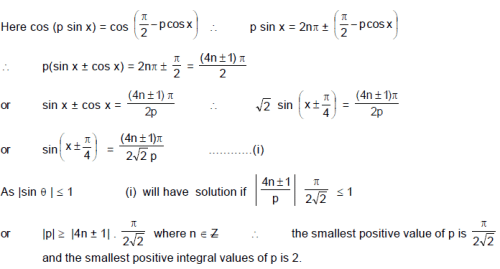*Answer can only contain numeric values
Part Test - 14 (JEE Advanced) - Question 60

Let image of the line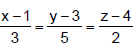in the plane 2x – y + z + 3 = 0 be L. A plane 7x + By + Cz + D = 0 is such that it contains the line L and is perpendicular to the plane 2x – y + z + 3 = 0, then find the value of D + 3C.

Detailed Solution for Part Test - 14 (JEE Advanced) - Question 60

Image Q of the point P(1, 3, 4) in the plane is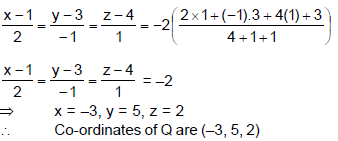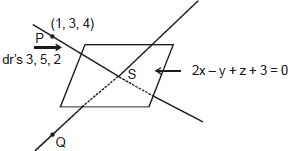Let S be the point of intersection of the given line & plane, then the co-ordinates of S are (3r + 1, 5r + 3, 2r + 4)
It lies on the plane
⇒ 2(3r + 1) – (5r + 3) + 2r + 4 + 3 = 0 ⇒ r = – 2
Hence, co-ordinate of 'S' are (–5, –7, 0)
therefore, equation of QS line is.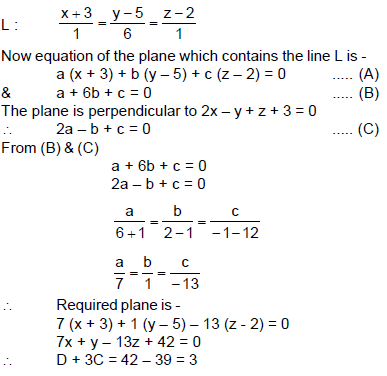Use Code STAYHOME200 and get INR 200 additional OFF Use Coupon Code